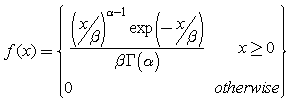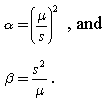# Gamma Statistical Distribution

The Gamma distribution is widely used in engineering, science, and business, to model continuous variables that are always positive and have skewed distributions. In UnWedge, the Gamma distribution can be useful for any variable which is always positive, such as cohesion or shear strength for example.

The Gamma distribution has the following probability density function:where G ( a ) is the Gamma function, and the parameters a and b are both positive (i.e., a > 0 and b > 0).

• a (alpha) is known as the shape parameter, while b (beta) is referred to as the scale parameter.
• b has the effect of stretching or compressing the range of the Gamma distribution. A Gamma distribution with b = 1 is known as the standard Gamma distribution.

The Gamma distribution represents a family of shapes. As suggested by its name, a controls the shape of the family of distributions. The fundamental shapes are characterized by the following values of a :

## Case I ( a < 1)

When a < 1, the Gamma distribution is exponentially shaped and asymptotic to both the vertical and horizontal axes.

## Case II ( a = 1)

A Gamma distribution with shape parameter a = 1 and scale parameter b is the same as an exponential distribution of scale parameter (or mean) b .

## Case III ( a > 1)

When a is greater than one, the Gamma distribution assumes a mounded (unimodal), but skewed shape. The skewness reduces as the value of a increases.

Examples of shapes of the standard Gamma distributions with different values of a are shown in the figure below.